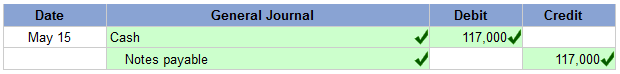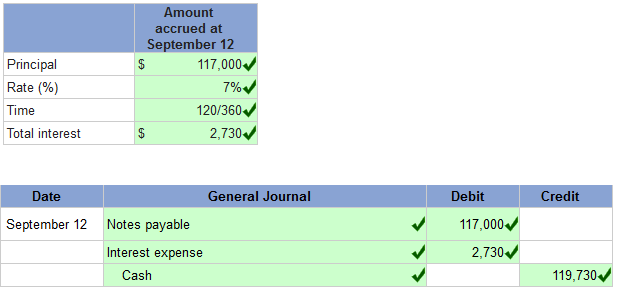Tuesday, 12 November 2013

Perfect Systems borrows \$117,000 cash on May 15, 2011, by signing a 120-day, 7% note. 1. On what date does this note mature? September 12, 2011 correct 2. Assume the face value of the note equals \$117,000, the principal of the loan. (a) Prepare the journal entries to record issuance of the note.

 Perfect Systems borrows \$117,000 cash on May 15, 2011, by signing a 120-day, 7% note.

 1. On what date does this note mature? September 12, 20112 Assume the face value of the note equals \$117,000, the principal of the loan.

 (a) Prepare the journal entries to record issuance of the note.(b)
First, complete the table below to calculate the interest expense at September 12. Use those calculated value to prepare your journal entries to record payment of the note at maturity. (Use 360 days a year. Do not round intermediate calculations.)Explanation: 1.
 Maturity date = May 15 + 120 days = September 12, 2011.

2(b)
 Calculation of Interest Expense

 Principal \$ 117,000 × Interest rate 7 % × Fraction of year 120/360 Total interest \$ 2,730